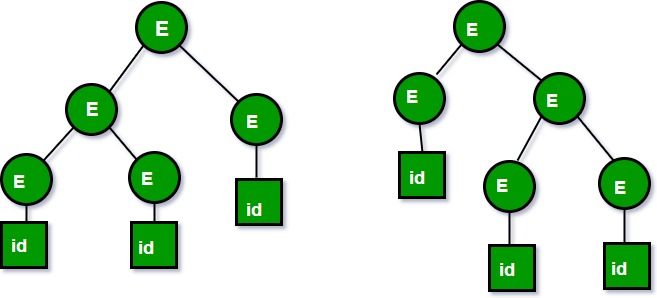# Ambiguous Grammar

• Difficulty Level : Easy
• Last Updated : 12 Nov, 2021

You can also read our previously discussed article on Classification of Context Free Grammars.

Context Free Grammars(CFGs) are classified based on:

• Number of Derivation trees
• Number of strings

Depending on Number of Derivation trees, CFGs are sub-divided into 2 types:

• Ambiguous grammars
• Unambiguous grammars

Ambiguous grammar:

A CFG is said to ambiguous if there exists more than one derivation tree for the given input string i.e., more than one LeftMost Derivation Tree (LMDT) or RightMost Derivation Tree (RMDT).

Definition: G = (V,T,P,S) is a CFG is said to be ambiguous if and only if there exist a string in T* that has more than one parse tree.
where V is a finite set of variables.
T is a finite set of terminals.
P is a finite set of productions of the form, A -> α, where A is a variable and α ∈ (V ∪ T)* S is a designated variable called the start symbol.

For Example:

1. Let us consider this grammar : E -> E+E|id

We can create 2 parse tree from this grammar to obtain a string id+id+id   :

The following are the 2 parse trees generated by left most derivation:Both the above parse trees are derived from same grammar rules but both parse trees are different. Hence the grammar is ambiguous.

2. Let us now consider the following grammar:

```Set of alphabets ∑ = {0,…,9, +, *, (, )}

E -> I
E -> E + E
E -> E * E
E -> (E)
I -> ε | 0 | 1 | … | 9

```

From the above grammar String 3*2+5 can be derived in 2 ways:

```I) First leftmost derivation                   II) Second leftmost derivation
E=>E*E                          E=>E+E
=>I*E                           =>E*E+E
=>3*E+E                                       =>I*E+E
=>3*I+E                           =>3*E+E
=>3*2+E                           =>3*I+E
=>3*2+I                           =>3*2+I
=>3*2+5                           =>3*2+5

```

Following are some examples of ambiguous grammars:

• S-> aS |Sa| Є
• E-> E +E | E*E| id
• A -> AA | (A) | a
• S -> SS|AB , A -> Aa|a , B -> Bb|b

Whereas following grammars are unambiguous:

• S -> (L) | a, L -> LS | S
• S -> AA , A -> aA , A -> b

Inherently ambiguous Language:

Let L be a Context Free Language (CFL). If every Context-Free Grammar G with Language L = L(G) is ambiguous, then L is said to be inherently ambiguous Language. Ambiguity is a property of grammar not languages. Ambiguous grammar is unlikely to be useful for a programming language, because two parse trees structures(or more) for the same string(program) implies two different meanings (executable programs) for the program.

An inherently ambiguous language would be absolutely unsuitable as a programming language, because we would not have any way of fixing a unique structure for all its programs.

For example,

`L = {anbncm} ∪ {anbmcm} `

Note : Ambiguity of grammar is undecidable, i.e. there is no particular algorithm for removing the ambiguity of grammar, but we can remove ambiguity by:

Disambiguate the grammar i.e., rewriting the grammar such that there is only one derivation or parse tree possible for a string of the language which the grammar represents.